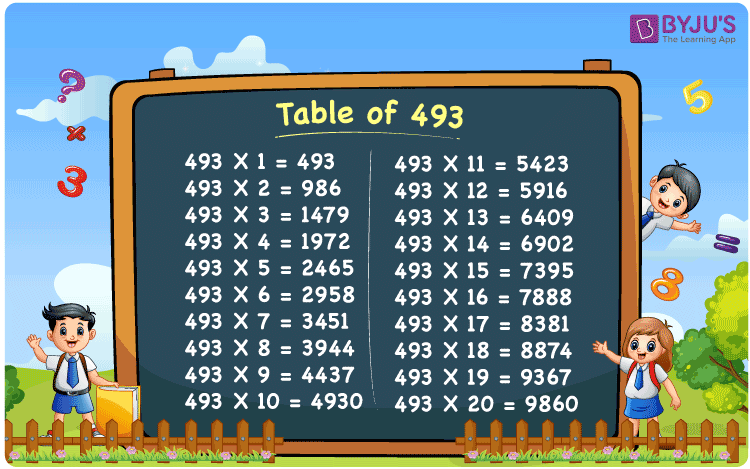Checkout JEE MAINS 2022 Question Paper Analysis : Checkout JEE MAINS 2022 Question Paper Analysis :

# Table of 493

The Table of 493 is provided in a tabular format to help students quickly check the values of multiples of 493. The multiplication table of 493 up to 20 is available in a PDF format to download, which will be handy while solving problems related to the 493 times table. The table of 493 will help while doing mental maths, which is a vital skill for appearing in any competitive examination.

## Table of 493 Chart

The 493 times table chart up to 20 is given here with an attractive image that can be used as a flashcard to learn the times table of 493. The multiplication table of 493 will help learners perform complex arithmetic calculations.## What is the 493 Times Table?

The 493 times table is the multiplication of 493 with any natural number. The 493 times table can be obtained by multiplication and also by adding 493 repeatedly. The below chart shows the multiplication table of 493, in both multiplication and addition form.

 493×1 = 493 493 493×2 = 986 493 + 493 = 986 493×3 = 1479 493 + 493 + 493 = 1479 493×4 = 1972 493 + 493 + 493 + 493 = 1972 493×5 = 2465 493 + 493 + 493 + 493 + 493 = 2465 493×6 = 2958 493 + 493 + 493 + 493 + 493 + 493 = 2958 493×7 = 3451 493 + 493 + 493 + 493 + 493 + 493 + 493 = 3451 493×8 = 3944 493 + 493 + 493 + 493 + 493 + 493 + 493 + 493 = 3944 493×9 = 4437 493 + 493 + 493 + 493 + 493 + 493 + 493 + 493 + 493 = 4437 493×10 = 4930 493 + 493 + 493 + 493 + 493 + 493 + 493 + 493 + 493 + 493 = 4930

## Multiplication Table of 493

Given below are the first 20 multiples of 493 that are obtained by the multiplication of 493 with the first 20 natural numbers.

 493 × 1 = 493 493 × 2 = 986 493 × 3 = 1479 493 × 4 = 1972 493 × 5 = 2465 493 × 6 = 2958 493 × 7 = 3451 493 × 8 = 3944 493 × 9 = 4437 493 × 10 = 4930 493 × 11 = 5423 493 × 12 = 5916 493 × 13 = 6409 493 × 14 = 6902 493 × 15 = 7395 493 × 16 = 7888 493 × 17 = 8381 493 × 18 = 8874 493 × 19 = 9367 493 × 20 = 9860

## Solved Examples on the Table of 493

Example 1:

What is two more than the third multiple of 493?

Solution:

The third multiple of 493 = 493 × 3 = 1479

Two more than 1479 = 1479 + 2 = 1481.

## Frequently Asked Questions on the Table of 493

### What are the times tables of 493?

The 493 times table is the multiplication of 493 with natural numbers. Starting with 493 × 1 = 493, 493 × 2 = 986, 493 × 3 = 1479, 493 × 4 = 1972, 493 × 5 = 2465 and so on.

### Which times table has 493?

493 comes in times tables of 1, 17, 29 and 493.

### How many times 17 is 493?

29 times 17, 29 × 17 = 493.